## autoML-FDR

This blog is about automating training of 500+ Flight data recorders(FDR) alarms using Machine Learning. On selection of certain hyperparameters, we get an analysis of which algorithm is best suited for training the dataset. This article explains the workflow of automating FDR, data pre processing, feature engineering and training. In the end, what challenges were faced while building this tool have been discussed, explaining how through autoML we can understand the challenges of the domain and provide useful insight for further advancing the state-of-the-art in several directions.

WHAT IS FDR?

FDR stands for Flight Data Recorder (essentially Failure Diagnostic Report) similar to the black box on airplanes. It is a device used to record specific aircraft performance parameters. The purpose of an FDR is to collect and record data from a variety of aircraft sensors onto a medium designed to survive an accident.The data essentially consists of a time series data of various data input.  Data includes  channels, transmit or receive, polarization, and min, max and value on a time series data.  The time series are hourly, minutes (during problems), and weekly (for older data when the system runs of storage space for log).

WORKFLOW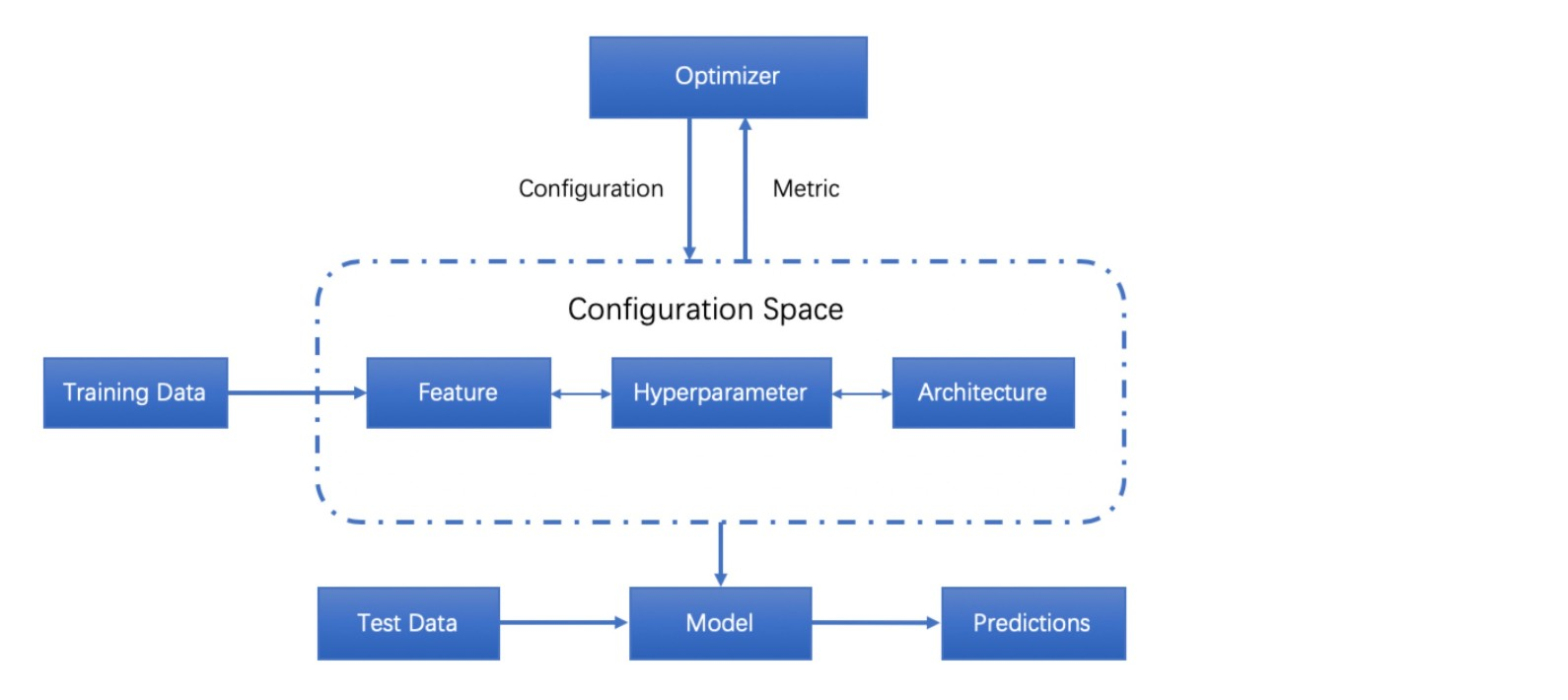OBJECTIVE

The overall objective is to find if an “anomaly” exists in the given FDR data, and indicate the “set of data” that are showing “non-normal” patterns.   Once the anomaly is detected, we will use “Grid Search” model to teach the system the root cause so in the future the probable causes can also be predicted.

FDR DATA OVERVIEW

This section describes the FDR data, data collection approach, data types, etc.,FDR data essentially collected by the system automatically on every sub system.  Here are the general attributes of the data elements:

• Can contain 1200 parameters to 9,000 or more parameters
• Same FDR data can have varying number of parameters - typically happens when firmware gets updated. Each section will have its own header that starts with Log Type
• Data are typically collected, for each module, as follows:
• For each channel - for example, 12 channels each with 100 GB
• Phases - polarizations - as information gets modulated on both X and Y (phase and amplitude)
• Min, Max and Value for each
• For example, the number of parameters could be 12 channels multiplied by 2 (RX/TX), multiplied by 4 (polarization) multiplied by 3 (min, max, val). These may or may not be consistent across observations.
• Various measurements for each channel further multiply the number of parameters being tracked; For example, for each channel, measurements may be for the last second, last hour, cumulative since last power mode (PM) is cleared
• To understand the anomaly pattern, it is important to detect the normal to abnormal behavior around a “given time”. The given time can be entered by the user as case open date may not be the correct time the problem indeed occurred. The analysis can look for a given time, go back backward to find hourly data which are typically “normal” operations and detect the parameters that are showing anomalies.
• FDR data are typically given in array form. The array index may not match the array in the firmware.  For example, the array index in firmware may be 0 based vs FDR data may be 1 based.
• Example: Q values → Tracking “Q” info is key to find anomaly.  The Q tracks the error rate before any error corrections are done.  The information is tracked in RX_FECQ data for all channels - last min, hour, and cumulative since last PM, and min, max and value for the respective time period.  For normal function the Q rate is between 6 and 12.  Anything less would indicate that the customers would be seeing errors in the data transmission.
• Within the FDR file, there are sections with varying numbers of columns. There exists a  mapping of column header to a parameter name outside the system.  For the initial phase, we will detect anomalies for the Q index
• While detecting the Q value anomaly, we need to consider if the board is going through start up process. During the start up process, the start code (e.g RX_STRT) starts from 100 goes to 0 which indicates the board/channel is “ready”. That should be the start where normal patterns should be observed.
• There is another code called DSP status which essentially indicates when a 5 sec interval data collection would start - essentially this is the time, there is some kind of anomaly in the board.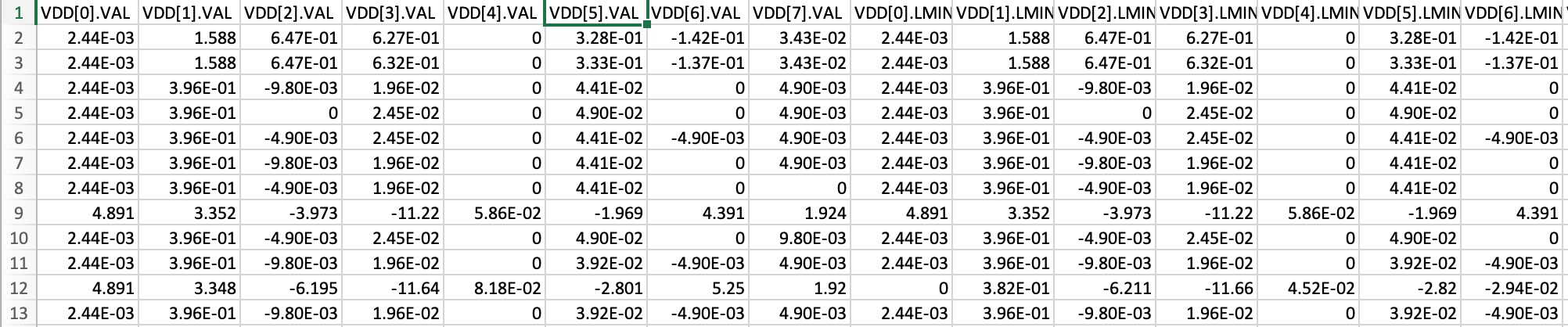DATA PRE PROCESSING

Firstly, the user inputs XML file name which has all the FDR alarm names, channel mapping file name that has all variable names mapped to channels(for user comprehension), alarm name on which the user wants the data to be trained on and FDR file name which consists the data for training. On submission, xml file is parsed and the data(DSP status) is segregated into 16 columns. This is an hexa data that shows which attributes the board thinks is a problem.  The diagram below shows the expansion of the hexa to the alarm codes: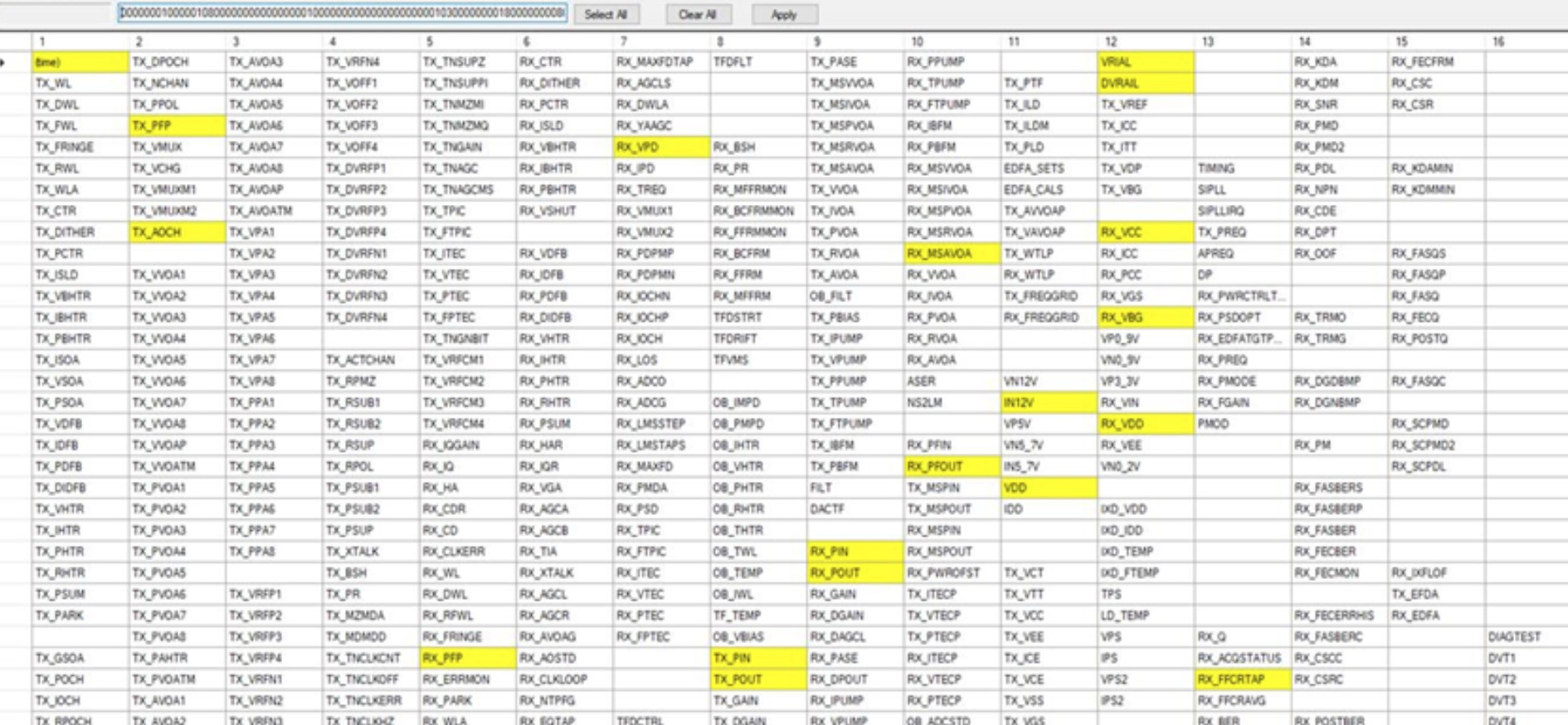the channel mappings file is parsed and two columns namely "LogType" and "Timestamp" are added to already existing STATUS columns. A dictionary is created where channel number is the key and dictionary values have all variable names corresponding to the keys.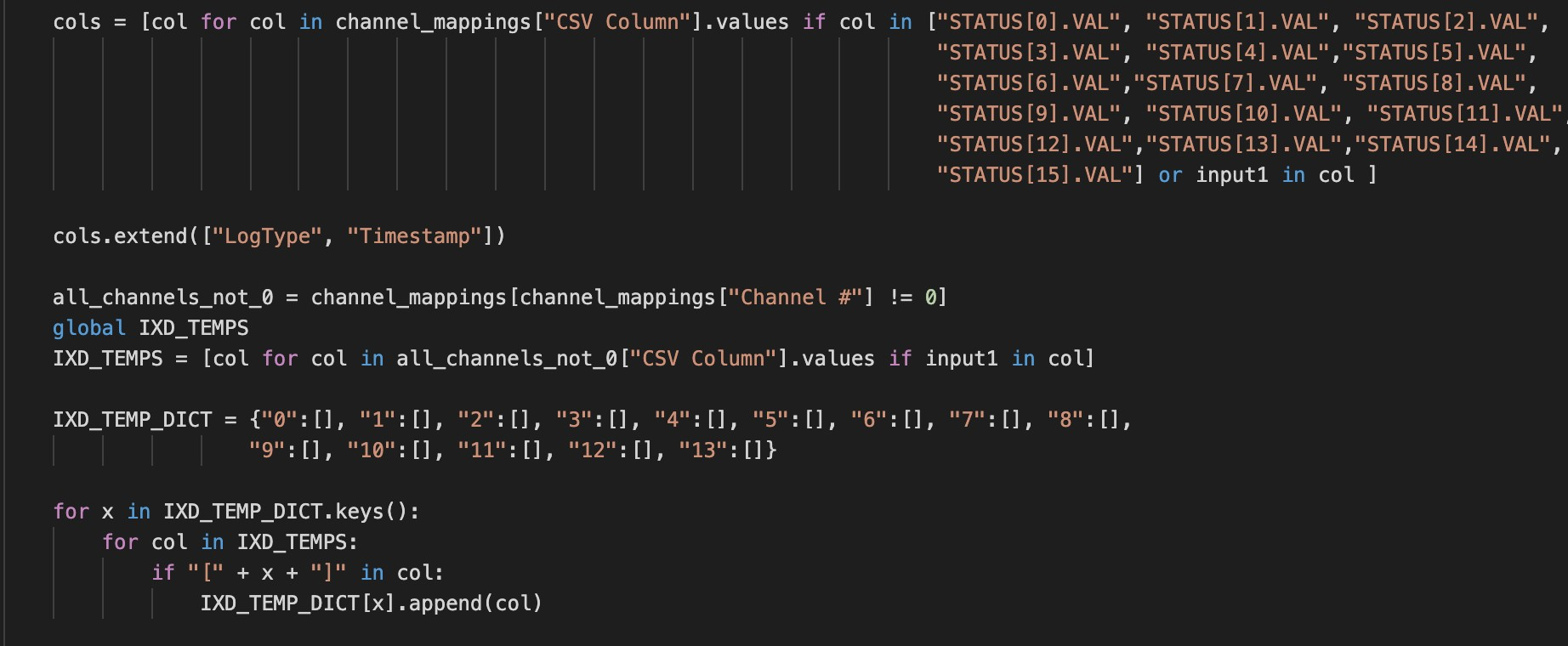All hexadecimal values are converted into binary values and data is divided between headerlist. The rows where new header list starts are treated as a separate dataset and intersection columns are calculated. These columns have the columns that are common in all the sub datasets calculated according to different header lists.

Now a dataframe of these values is created.Alarm name entered by the user id searched in the xml file and  column number where it lies is calculated. All XML file columns are divided into seven sets of four alarms each. The set where that particular alarm lies is mapped to  atruth table and an "index" column is added to it.

DATA PREPARATION(FEATURE ENGINEERING)

Positive and Negative samples are calculated. Pure positive samples contain the indices where only the input alarm has value 1(means the alarm is set). Positive samples with other alarm has samples where the input alarm along with other alarms in the truth table has value 1. Pure negative samples has indices where only the input alarm has value 0(means the alarm is not set). Negative samples with other alarms has indices where input alarm along with other alarms has value 0.

This is how Pure Negative samples look like: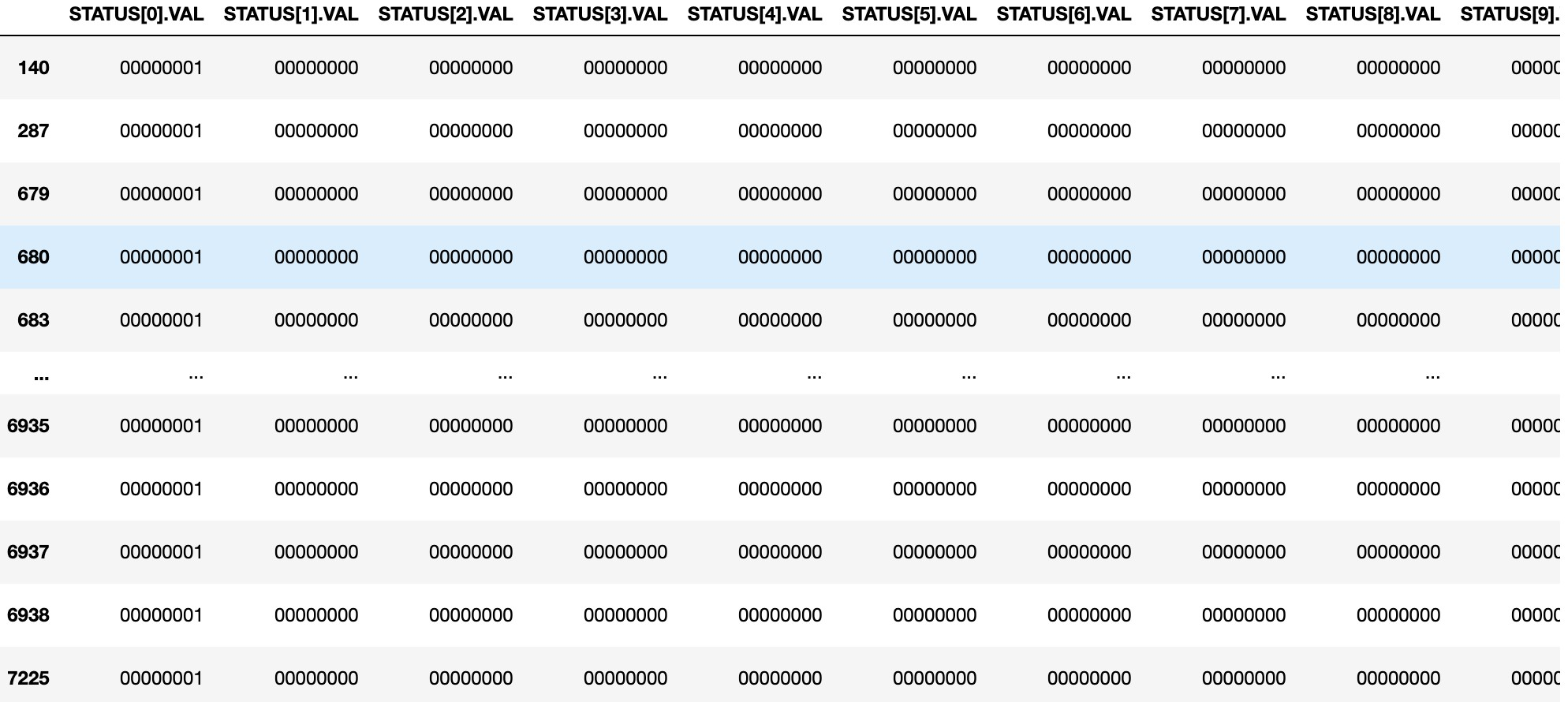Negative Samples with other alarms look like: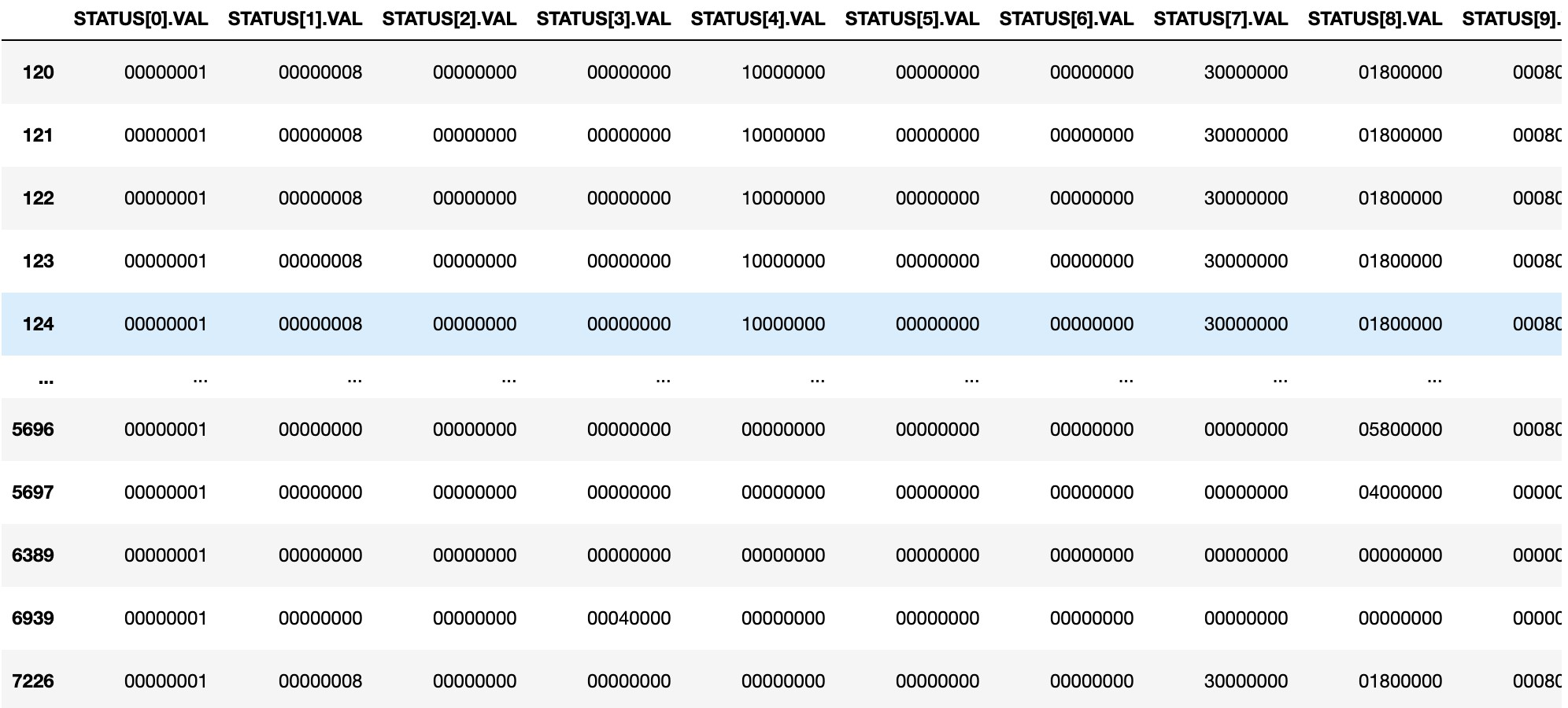now the user inputs the channel number in the dictionary that has the problem. Here channel number is the key and MIN, MX and VAL are the values. Now all the dataframes of positive and negative samples are filtered according to the channel number entered by the user. the channel number taken as an input is added to the positive dataset and rest of the values are added to the negative dataset.

Now all positive samples (pure positives and positives with other alarms) are added and all negative samples(pure negatives and negatives with other alarms).

Pure Negative dataframe where input alarm is present is described below.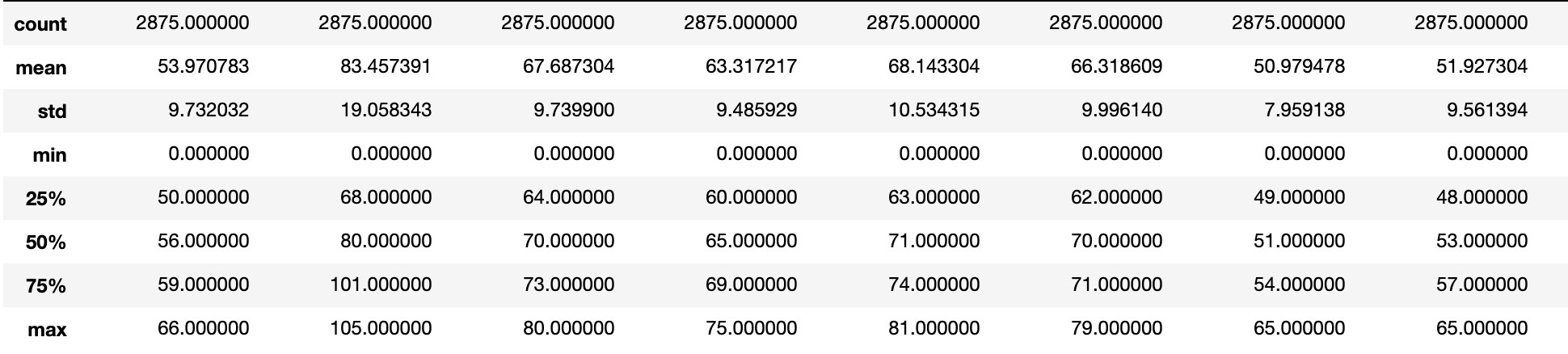Now time series data that is 'correlated' in nature is split into sequences, this is called "Moving Average".Here next observation is mean of all past observations. A window of range 5 is taken to define moving average model. This will smooth time series and highlight different trends. The data has lags(assumed to be 4) and mean, min and max values are calculated. This is done for both negative and positive samples. These positive samples show where the anomaly is and negative samples show where there's no anomaly.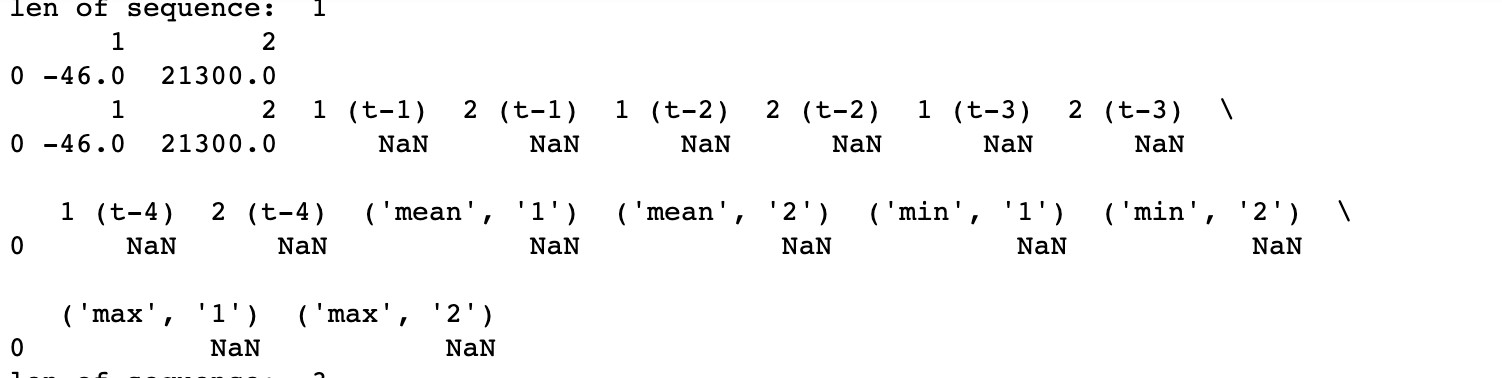Finally the model is trained using Grid Search with multiple classifiers. Various hyperparameters are chosen w.r.t the classifier used. Hyper-parameters are input into any machine learning model which generates its own parameters in order to influence the values of said generated parameters in the hope of making the model more accurate.Listed below are various classifiers and their respective hyperparameters used.

1)Logistic Regression

• Penalty: is used to specify the method of penalization of the coefficients of noncontributing variables.
• Lasso (L1) performs feature selection as it shrinks the less important feature’s coefficient to zero.
• Ridge (L2) all variables are included in model, though some are shrunk. Less computationally intensive than lasso.
• C: is the inverse of the regularization term (1/lambda). It tells the model how much large parameters are penalized, smaller values result in larger penalization; must be a positive float.
• Common values: [0.001,0.1 …10..100]
• class_weight: allows you to place greater emphasis on a class. For example, if the distribution between class 1 and class 2 is heavily imbalanced, the model can treat the two distributions appropriately.
• Default is that all weights = 1. Class weights can be specified in a dictionary.
• “Balanced” will create class weights that are inversely proportional to class frequencies, giving more weight to individual occurrences of smaller classes.

2)Linear Regression

• Fit intercept: specifies whether the intercept of the model should be calculated or set to zero.
• If false, intercept for regression line will be 0.
• If true, model will calculate the intercept.
• Normalize: specifies whether to normalize the data for the model using the L2 norm.

3)SVM

• C: is the inverse of the regularization term (1/lambda). It tells the model how much large parameters are penalized, smaller values result in larger penalization; must be a positive float.
• A higher C will cause the model to misclassify less, but is much more likely to cause overfit.
• Good range of values : [0.001, 0.01, 10, 100, 1000…]
• class_weight: Set the parameter of class i to be class_weight[i] *C.
• This allows you to place greater emphasis on a class. For example, if the distribution between class 1 and class 2 is heavily imbalanced, the model can treat the two distributions appropriately.
• Default is that all weights = 1. Class weights can be specified in a dictionary.
• “Balanced” will create class weights that are inversely proportional to class frequencies, giving more weight to individual occurrences of smaller classes.

4)K-Nearest Neighbours

• n_neighbors: determines the number of neighbors used when calculating the nearest-neighbors algorithm.
• Good range of values: [2,4,8,16]
• p: power metric when calculating the Minkowski metric, a fairly mathematically complex topic. When evaluating models, simply trying both 1 and two here is usually sufficient.
• Use value 1 to calculate Manhattan distance
• Use value 2 to calculate Euclidean distance (default)

5)Random Forest

• n_estimators: sets the number of decision trees to be used in the forest.
• Default is 100
• Good range of values: [100, 120, 300, 500, 800, 1200]
• max_depth: Set the max depth of the tree.
• If not set then there is no cap. The tree will keep expanding until all leaves are pure.
• Limiting the depth is good for pruning trees to prevent over-fitting on noisy data.
• Good range of values: [5, 8, 15, 25, 30, None]
• min_samples_split: The minimum number of samples needed before a split (differentiation) is made in an internal node
• Default is 2
• Good range of values: [1,2,5,10,15,100]
• min_samples_leaf: The minimum number of samples needed to create a leaf (decision) node.
• Default is 1. This means that a split point at any depth will only be allowed if there is at least 1 sample for each path.
• Good range of values: [1,2,5,10]
• max_features: Set the number of features to consider for the best node split
• Default is “auto”, which means that the square root of the number of features is used for every split in the tree.
• “None” means that all features are used for each split.
• Each decision tree in the random forest will typically use a random subset of features for splitting.
• Good range of values: [log2, sqrt, auto, None]

Training accuracy of all the classifiers for the dataset is calculated and analysed. For this dataset, Logistic Regression gave the highest accuracy.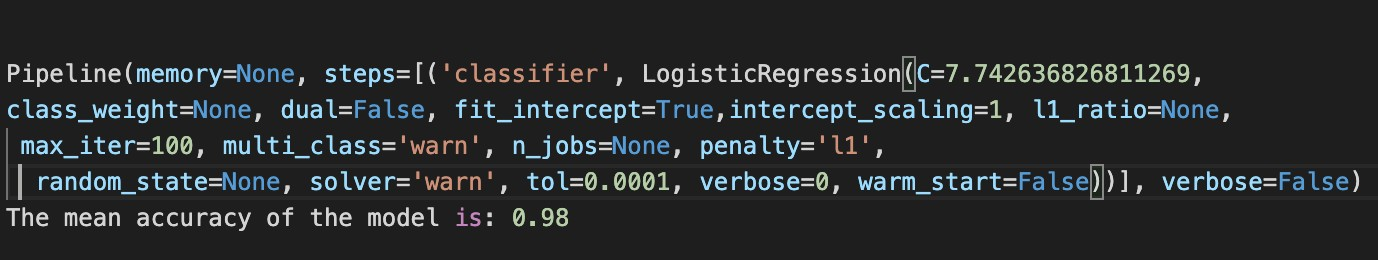Confidence of input alarm is visulaized below: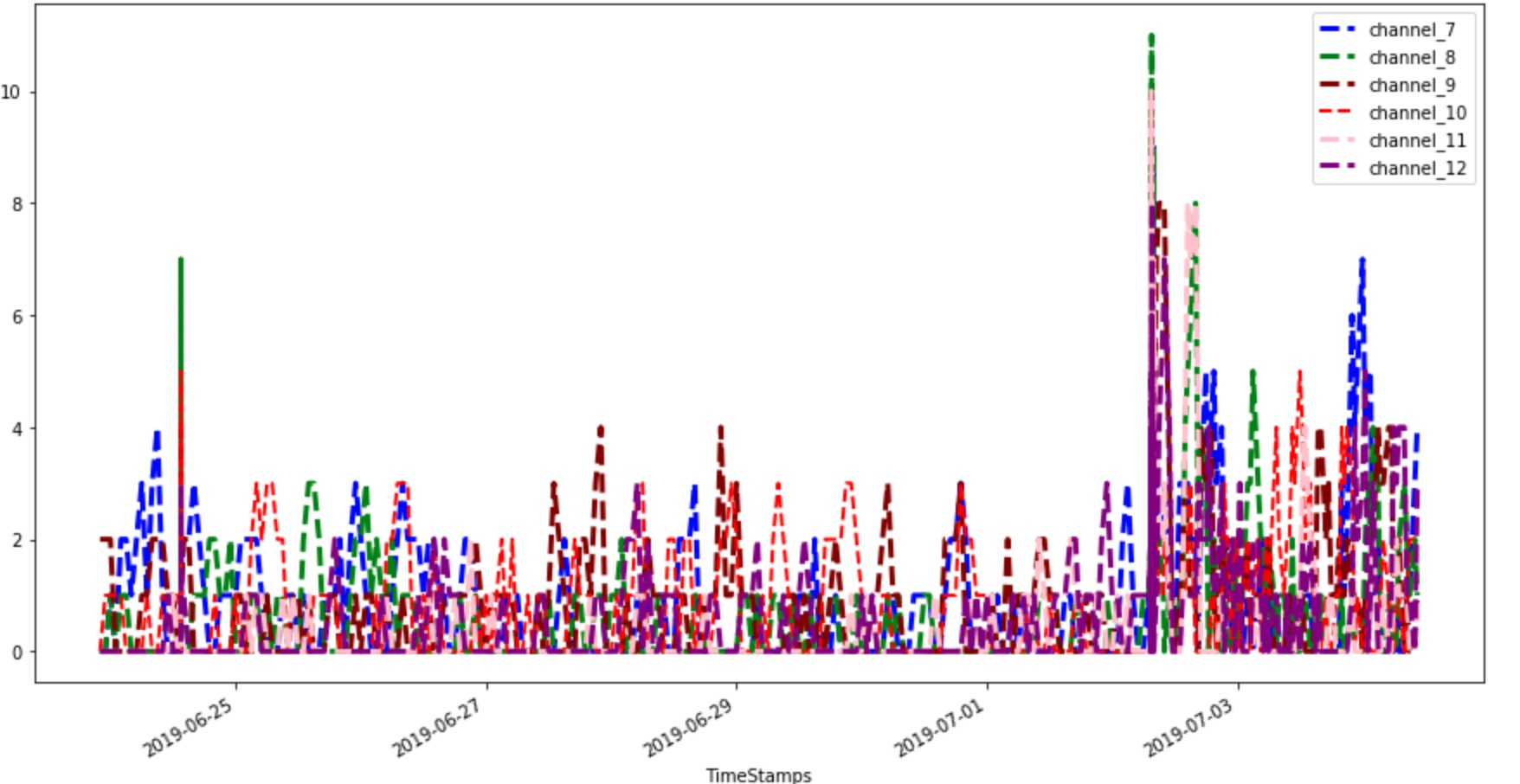OPEN CHALLENGES AND FUTURE DIRECTIONS

• DATA PREPARATION:: In practice, there is a crucial need for automating the feature extraction process as it is considered as one of the most time consuming part of the pipeline. In practice, most of the systems neglect the automation of transferring data features into different domain space like performing principal component analysis, or linear discriminant analysis and when they improve the model performance. So, further research is needed to try different architectures and interpret them to have the ability to automate the choice of suitable encoders. Furthermore, there are various techniques for measuring a score for the feature importance which is a very important part to automate the feature selection process. However, there is no comprehensive comparative studies between these methods or good recipes that can recommend when to use each of these techniques.
• DATA VALIDATION: In this context, most of the solutions in literature focus on problem detection and user notification only. However, automatic correction hasn’t been investigated in a good manner that covers several possible domains of datasets and reduce the data scientist’s role in machine learning production pipeline. In addition, as the possible data repairing is a NP-Hard problem, there is a need to find more approximation techniques that can solve this problem.
• USER FRIENDLINESS:In general, most of the current tools and framework can not be considered to be user friendly. They still need sophisticated technical skills to be deployed and used. Such challenge limits its usability and wide acceptance among layman users and domain experts (e.g., physicians, accountants) who commonly have limited technical skills. Providing an interactive and light-weight web interfaces for such framework can be one of the approaches to tackle these challenges.

CONCLUSION

Automating this process using machine Learning helped to reduce man hours of training time by 16 hours.  AutoML will help in implementing ML solutions that do not require extensive programming knowledge, leverage data science best practices, provide agile problem-solving skills, and most importantly, help save time and computing resources.

REFERENCES

1. 2019 | AutoML: A Survey of the State-of-the-Art | Xin He.           https://arxiv.org/pdf/1908.00709.pdf
2. 2019 | Automated Machine Learning: State-of-The-Art and Open Challenges | Radwa Elshawi.  https://arxiv.org/pdf/1906.02287.pdf
3. 2017 | AutoLearn — Automated Feature Generation and Selection | Ambika Kaul, et al. | ICDM |.   https://ieeexplore.ieee.org/document/8215494
4. 2016 | Automating Feature Engineering | Udayan Khurana, et al. | NIPS |.  http://workshops.inf.ed.ac.uk/nips2016-ai4datasci/papers/NIPS2016-AI4DataSci_paper_13.pdf

Search
Categories